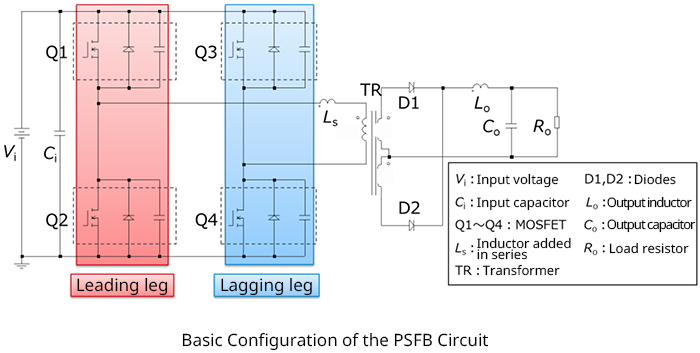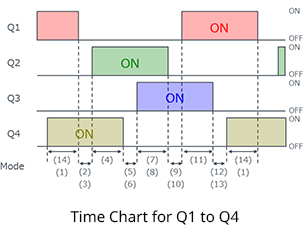Technical Information Site of Power Supply Design

2021.09.15 Si Power Device

# Basic Configuration of a PSFB Circuit

Improving the Power Conversion Efficiency of Phase Shift Full Bridge Circuits

Introduction

As our second installment relating to evaluation of Si power devices, we here begin a series of articles relating to "Improving the Power Conversion Efficiency of Phase Shift Full Bridge Circuits".

In recent years, ever-greater power output has been demanded from power supplies for servers, onboard chargers, and other equipment. In many such high-level power supplies, full bridge circuits are used; in particular, phase shift full bridge (hereafter "PSFB") circuits are capable of zero-voltage switching ("ZVS") at turn-on of switching elements such as super-junction MOSFETs (SJ MOSFETs) and IGBTs for reduced switching losses, and hence can handle even higher power levels.

As the power capacities of power supplies have risen, improvement of efficiency has become an urgent issue. In general, even when efficiency is the same, losses themselves are larger in a power supply handling large amounts of power, and so the highest possible efficiency is sought.

In this section, the need for a fast-recovery type SJ MOSFET when using an SJ MOSFET in a PSFB circuit is explained in terms of the circuit operation. The efficiencies resulting for SJ MOSFETs with different recovery characteristics are compared, and the importance of the recovery characteristic in a PSFB circuit is confirmed.

Basic Configuration of a PSFB Circuit

To begin with, the basic configuration of a PSFB circuit is presented. Upon grasping this basic configuration, the circuit operation, described below, should also be easy to understand.The leakage inductance of the transformer may be utilized as a resonance inductor in order to realize ZVS, which is one feature of the PSFB configuration; however, in some cases an inductor is added in series with the transformer to broaden the range of ZVS operation. Here, a circuit is assumed in which an added series inductor LS is used.

Next, the on/off timing chart for the switches is shown. The figures appearing in the bottom below the bidirectional arrows are labels representing the circuit operation modes. These correspond to the mode numbers used in the following explanation of circuit operation.

As shown in the chart, after the on/off states of Q1 and Q2 have been swapped, the on/off states of Q3 and Q4 are swapped with a certain phase lag. From this fact, the leg of Q1 and Q2 is generally called the "leading leg", and the leg of Q3 and Q4 is the "lagging leg".#### Key Points:

・Phase shift full bridge (PSFB) circuits make possible dramatic reductions in switching losses through zero-voltage switching (ZVS) operation of switching elements, and so can handle greater amounts of power.

・A PSFB switching circuit essentially consists of four switching elements (MOSFETs); the leakage inductance of a transformer is used as a resonance inductance necessary for ZVS operation.

・In order to extend the range of ZVS operation, there are cases in which an inductor is added in series. Here, a circuit with an added series inductor is assumed.

・In the basic switching operation, after the on/off states of Q1 and Q2 have been swapped, the on/off states of Q3 and Q4 are swapped after a certain phase lag.

・In general, the leg of Q1 and Q2 is called the "leading leg", and the leg of Q3 and Q4 is the "lagging leg".